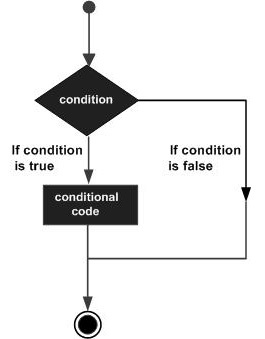# Elm - Decision Making

#### Complete Python Prime Pack for 2023

9 Courses     2 eBooks

#### Artificial Intelligence & Machine Learning Prime Pack

6 Courses     1 eBooks

#### Java Prime Pack 2023

8 Courses     2 eBooks

Decision-making structures requires that the programmer specifies one or more conditions to be evaluated or tested by the program, along with a statement or statements to be executed if the condition is determined to be true, and optionally, other statements to be executed if the condition is determined to be false.

Shown below is the general form of a typical decision-making structure found in most of the programming languagesA decision-making construct evaluates a condition before the instructions are executed. Decision-making constructs in Elm are classified as follows −

Sr. No. Statement Description
1 if...then...else statement The if statement consists of a Boolean expression followed by then which is executed if the expression returns true and else which is executed if the expression returns false
2 nested if statement You can use one if...then...else inside another if.
3 case statement Tests the value of a variable against a list of values.

## if...then...else Statement

The if…then construct evaluates a condition before a block of code is executed. If the Boolean expression evaluates to true, then the block of code inside the then statement will be executed. If the Boolean expression evaluates to false, then the block of code inside the else statement will be executed.

Unlike other programming languages, in Elm we must provide the else branch. Otherwise, Elm will throw an error.

### Syntax

```if boolean_expression then statement1_ifTrue else statement2_ifFalse
```

### Illustration

Try the following example in the REPL terminal.

```> if 10>5 then "10 is bigger" else "10 is small"
"10 is bigger" : String
```

## Nested If

The nested if statement is useful for testing multiple conditions. The syntax of a nested if statement is given below −

```if boolean_expression1 then statement1_ifTrue else if boolean_expression2 then statement2_ifTrue else statement3_ifFalse
```

### Illustration

Try the following example in the Elm REPL −

```> score=80
80 : number
> if score>=80 then "Outstanding" else if score > = 70 then "good" else "average"
"Outstanding" : String
```

## Case statement

The case statement can be used to simplify the if then else statement. The syntax of a case statement is as given below −

```case variable_name of
constant1 -> Return_some_value
constant2 -> Return_some_value
_ -> Return_some_value if none of the above values match
```

The case statement checks if the value of a variable matches a predefined set of constants and returns the corresponding value. Note that value returned by each case must be of the same type. If the variables value does not match any of the given constants, the control is passed to * default * (denoted by //_ ) and the corresponding value is returned.

### Illustration

Try the following example in the Elm REPL −

```> n = 10
10 : number
> case n of \
| 0 -> "n is Zero" \
| _ -> "n is not Zero"
"n is not Zero" : String
```

The above code snippet checks if the value of n is zero. The control is passed to default, which returns the string “n is not Zero”.